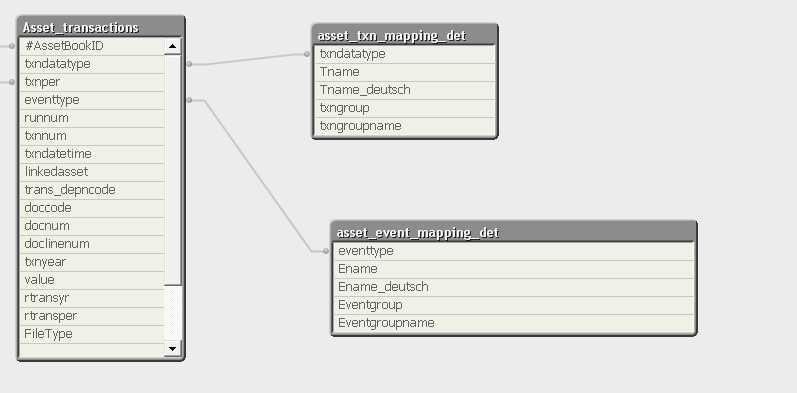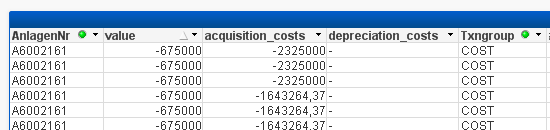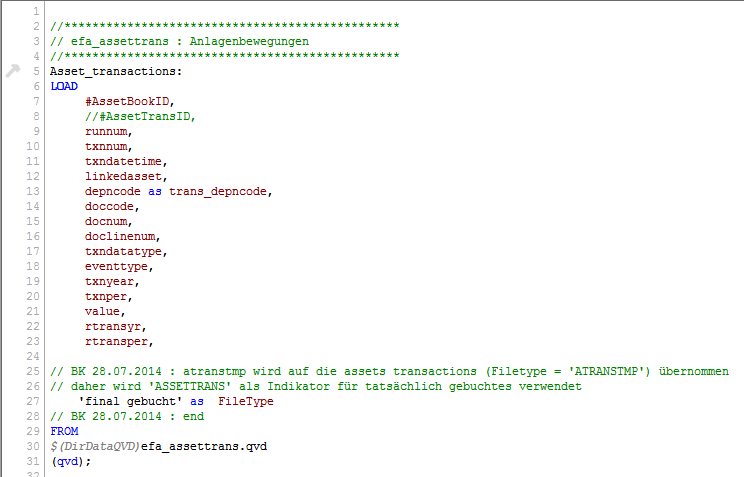# QlikView Creating Analytics

Discussion Board for collaboration related to Creating Analytics for QlikView.Not applicable

## Formula in Load Script

Hi everybody,

I hope someone of you can help me.

I have a table with asset Transactions. Now I have to separate the field value in the script.

i.e.

If(txngroup='COST', value) as acquisition_costDoes someone of you maybe have an idea?

Thanks

Chris

1 Solution

Accepted SolutionsPartner

## Re: Formula in Load Script

Hi Chris,

I would say you are nearly there.

I would advise you keep the  AssetTransID as this seems to be the key field.

If you then want a combine cost be assets you would want a aggrate table something like

ValueLoad:

Load

AssetBookID

IF(Txngroup='Cost', Sum(Value)     as acquistion_costs,

...

..

Resident Asset_transactions

Group by AssetBookID;

Mark

7 RepliesMVP

## Re: Formula in Load Script

Hi Chris,

For this you will have to join the "Asset Transaction Mapping Det" Table with "Asset Transaction" Table first and then after join you can use resident load to write your condition, same as what you have written.

Regards,

Kaushik SolankiPartner

## Re: Formula in Load Script

Hi,

You can able to do by 2 ways

1) create a mapping file

TXNGROUP_Mapping:

mapping

load

txndatatype,

txngroup

resident asset_txn_mapping_det where txngroup = 'COST';

2) use apply map function

if(applymap('TXNGROUP_Mapping',txndatatype,0) = 'COST', value) as acquisition_cost

in Asset_Transaction table

-Sathish

HighlightedPartner

## Re: Formula in Load Script

another way:

use left join with asset_txn_mapping_det to Asset_Transaction table

after that,

Asset_Transaction table_Temp:

load

*,

If(txngroup='COST', value) as acquisition_cost

resident Asset_Transaction table;

drop table Asset_Transaction table;

-SathishNot applicable

## Re: Formula in Load Script

Hi everybody,

thanks for the quick Responses. I chose to use the left join but this does not work.

But I have two Problems maybe due to same reason:

The field Value has -675000, due to txngroup = 'COST', the field acquistion_costs should also have -675000, but has -2325000

The field depreciation_costs should have a Zero (0,00)the Field Value has -11250, due to txngroup = 'DEPN' the field acquisition_costs should have a Zero, but has a value in it.

The field depreciation_costs is null but should have the same entry as the field value (-11250)What is my mistake? How can I get this to work?

This is my Script:Thanks

ChrisPartner

## Re: Formula in Load Script

Hi Chris,

I would say you are nearly there.

I would advise you keep the  AssetTransID as this seems to be the key field.

If you then want a combine cost be assets you would want a aggrate table something like

ValueLoad:

Load

AssetBookID

IF(Txngroup='Cost', Sum(Value)     as acquistion_costs,

...

..

Resident Asset_transactions

Group by AssetBookID;

MarkPartner

## Re: Formula in Load Script

HI,

"AnlageNR" field is coming from which table? what is the link between "AnlageNR" field table and Value load?

-Sathsih

Contributor III

## Re: Formula in Load Script

Hello Christian,

AssetTransaction:

LOAD

#AssetBookID,

txndatatype as DataTypeID,

eventtype as EventTypeID,

value

FROM efa.assettrans.qvd;

AssetTransactionGroup:

LOAD

Tcode as DataTypeID,

IF(Txngroup = 'COST', 'AquisitionCost',

IF(Txngroup = 'DEPN', 'DepreciationCost',

IF(Txngroup = 'DISP', 'Disposals',

IF(Txngroup = 'TRA', 'Transfers', 'UnknownTransGroup') ))) as TransactionGroup

FROM assets_mapping.xlsx;

AssetEventGroup:

LOAD

Ecode as EventTypeID,

IF(match(EventGroup, 'DEPN', 'ADD', 'DEPC'), 'Addition', 'UnknownEventGroup') as EventGroup

FROM assets_mapping.xlsx;

Using a Set analysis in CHARTS, you can calculate any of your Group's values, ex.:

Sum( {<TransactionGroup = {'COST'}>} Value) as AquisitionCosts,

Sum( {<TransactionGroup = {'DEPN'}>} Value) as DepreciationCosts;

In table box use your desired fields.

Of course, fields could be prepared the way you mentioned in script by creation separated field values, but joins would be heavy operations.

Best regards,

Martin